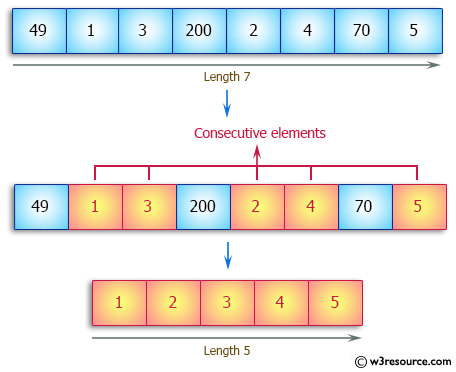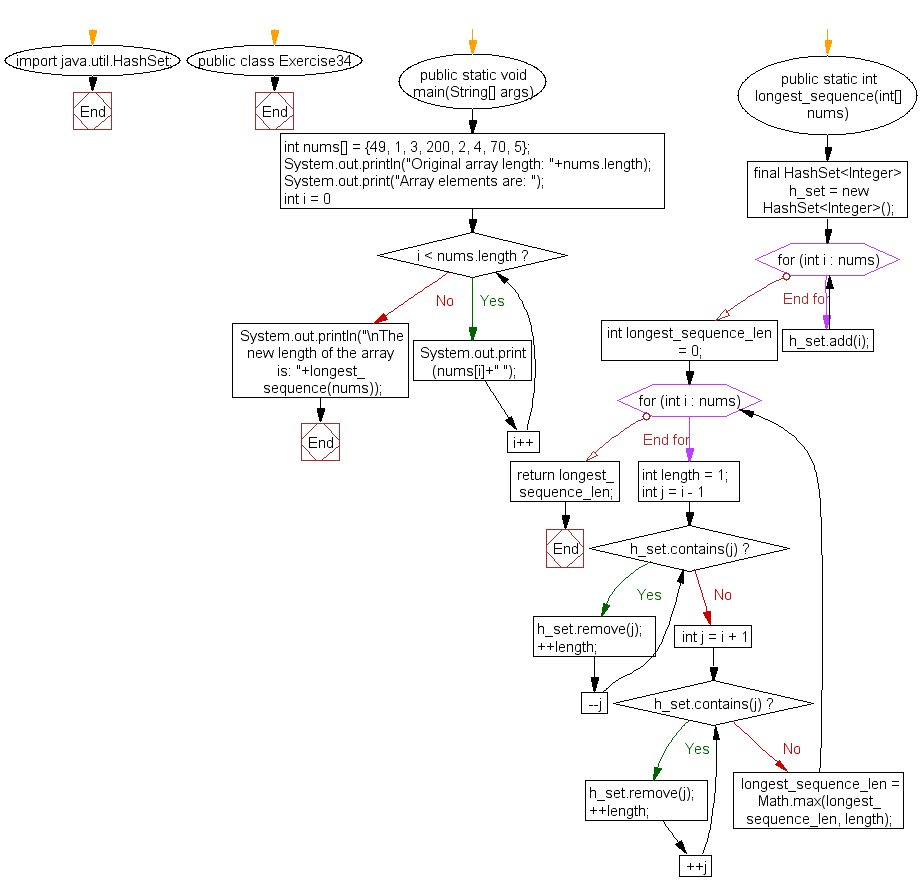﻿ Java: Longest sequence of elements from an unsorted array# Java Array Exercises: Find the length of the longest consecutive elements sequence from a given unsorted array of integers

## Java Array: Exercise-34 with Solution

Write a Java program to find the length of the longest consecutive elements sequence from a given unsorted array of integers.
Sample array: [49, 1, 3, 200, 2, 4, 70, 5]
The longest consecutive elements sequence is [1, 2, 3, 4, 5], therefore the program will return its length 5.

Pictorial Presentation:Sample Solution:

Java Code:

``````import java.util.HashSet;
public class Exercise34 {
public static void main(String[] args) {
int nums[] = {49, 1, 3, 200, 2, 4, 70, 5};
System.out.println("Original array length: "+nums.length);
System.out.print("Array elements are: ");
for (int i = 0; i < nums.length; i++)
{
System.out.print(nums[i]+" ");
}
System.out.println("\nThe new length of the array is: "+longest_sequence(nums));

}

public static int longest_sequence(int[] nums) {
final HashSet<Integer> h_set = new HashSet<Integer>();
for (int i : nums) h_set.add(i);

int longest_sequence_len = 0;
for (int i : nums) {
int length = 1;
for (int j = i - 1; h_set.contains(j); --j) {
h_set.remove(j);
++length;
}
for (int j = i + 1; h_set.contains(j); ++j) {
h_set.remove(j);
++length;
}
longest_sequence_len = Math.max(longest_sequence_len, length);
}
return longest_sequence_len;
}
}
```
```

Sample Output:

```
Original array length: 8
Array elements are: 49 1 3 200 2 4 70 5
The new length of the array is: 5
```

Flowchart:Visualize Java code execution (Python Tutor):

Java Code Editor:

Improve this sample solution and post your code through Disqus

What is the difficulty level of this exercise?

Test your Programming skills with w3resource's quiz.

﻿

## Java: Tips of the Day

countOccurrences

Counts the occurrences of a value in an array.

Use Arrays.stream().filter().count() to count total number of values that equals the specified value.

```public static long countOccurrences(int[] numbers, int value) {
return Arrays.stream(numbers)
.filter(number -> number == value)
.count();
}
```

Ref: https://bit.ly/3kCAgLb